# Arduino Radar with Led diode and encoding data onto an image

Practical development of the radar system for detection of objects at short distances and encoding data onto an image using QuickStego tool.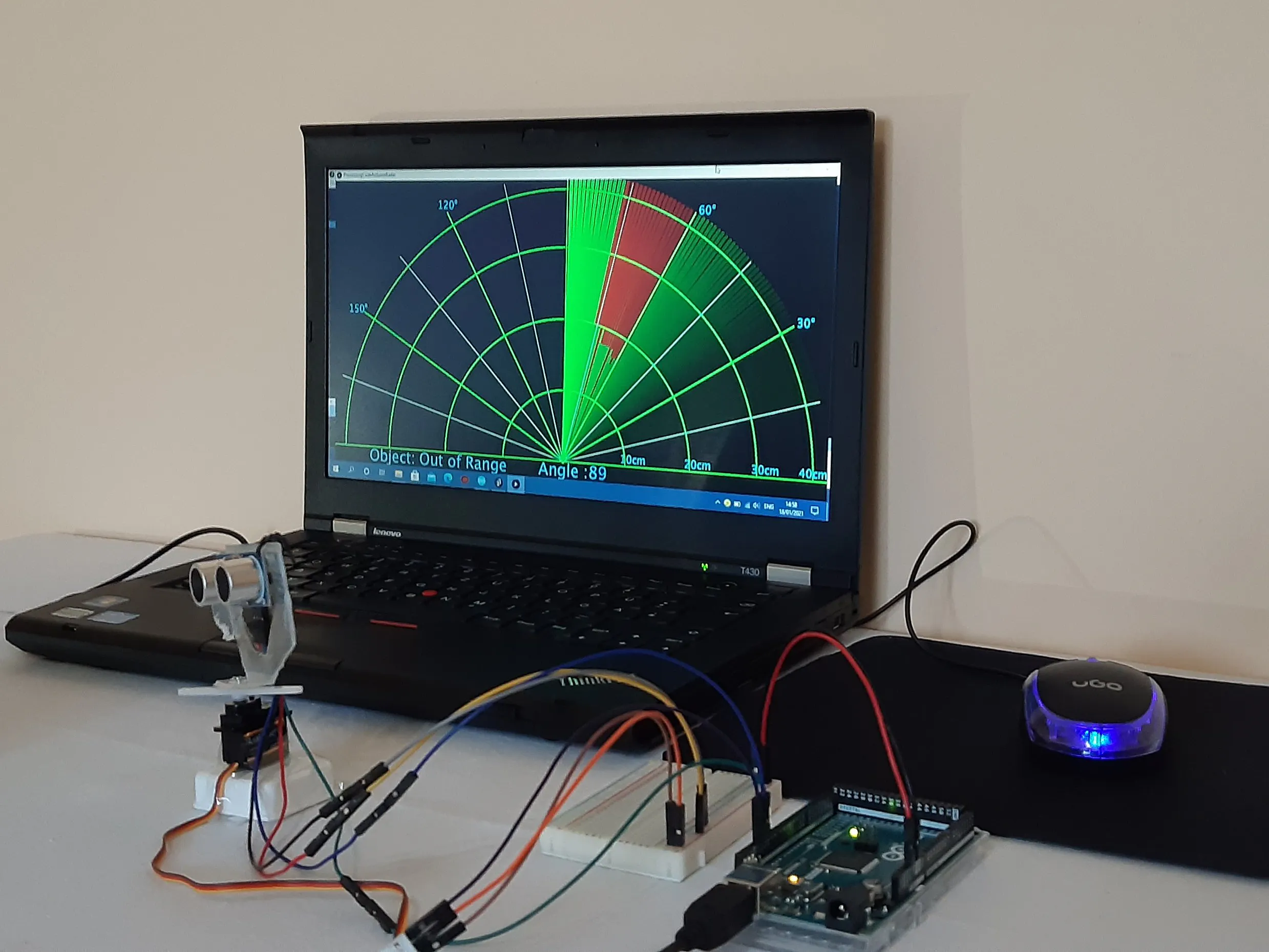## Things used in this project

### Hardware components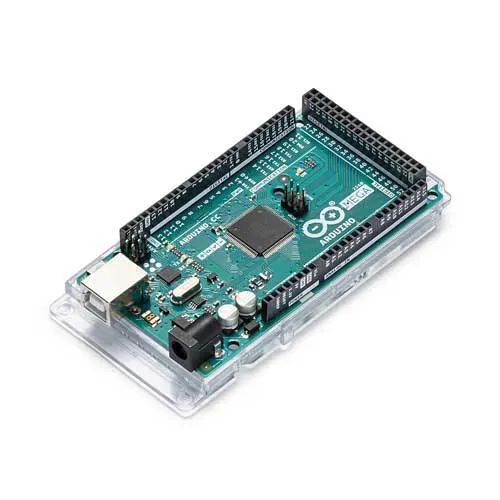Arduino Mega 2560
×1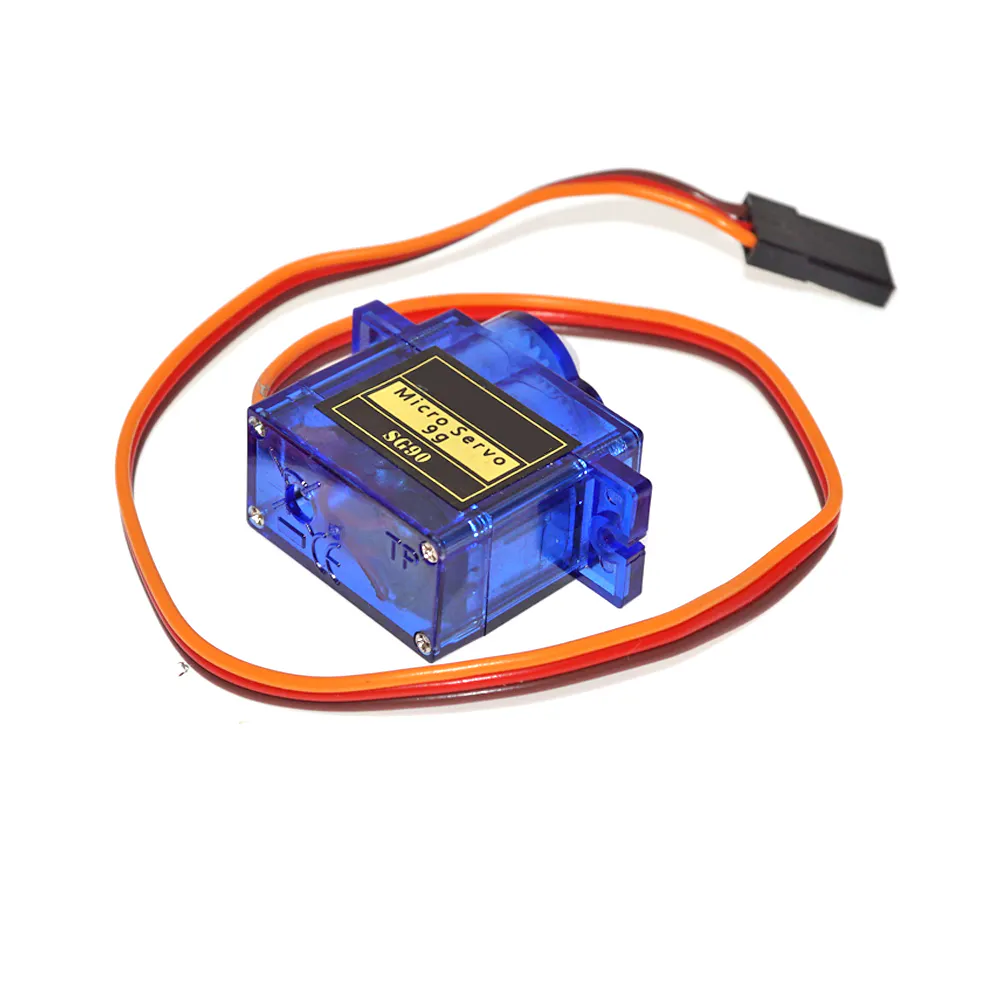SG90 Micro-servo motor
×1Ultrasonic Sensor - HC-SR04 (Generic)
×1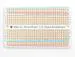×1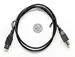USB-A to B Cable
×1LED (generic)
×1
 Amazon Web Services Holder for Ultrasonic Sensor HC-SR04
×1

### Software apps and online servicesArduino IDE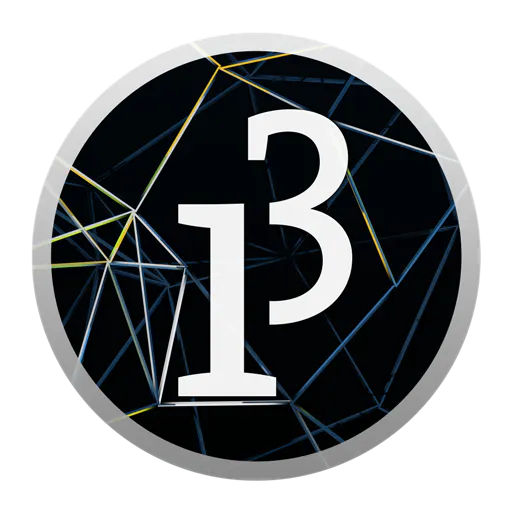The Processing Foundation Processing

## Schematics

### SCHEMATICS

Arduino Mega 2560 Sketch of connecting the components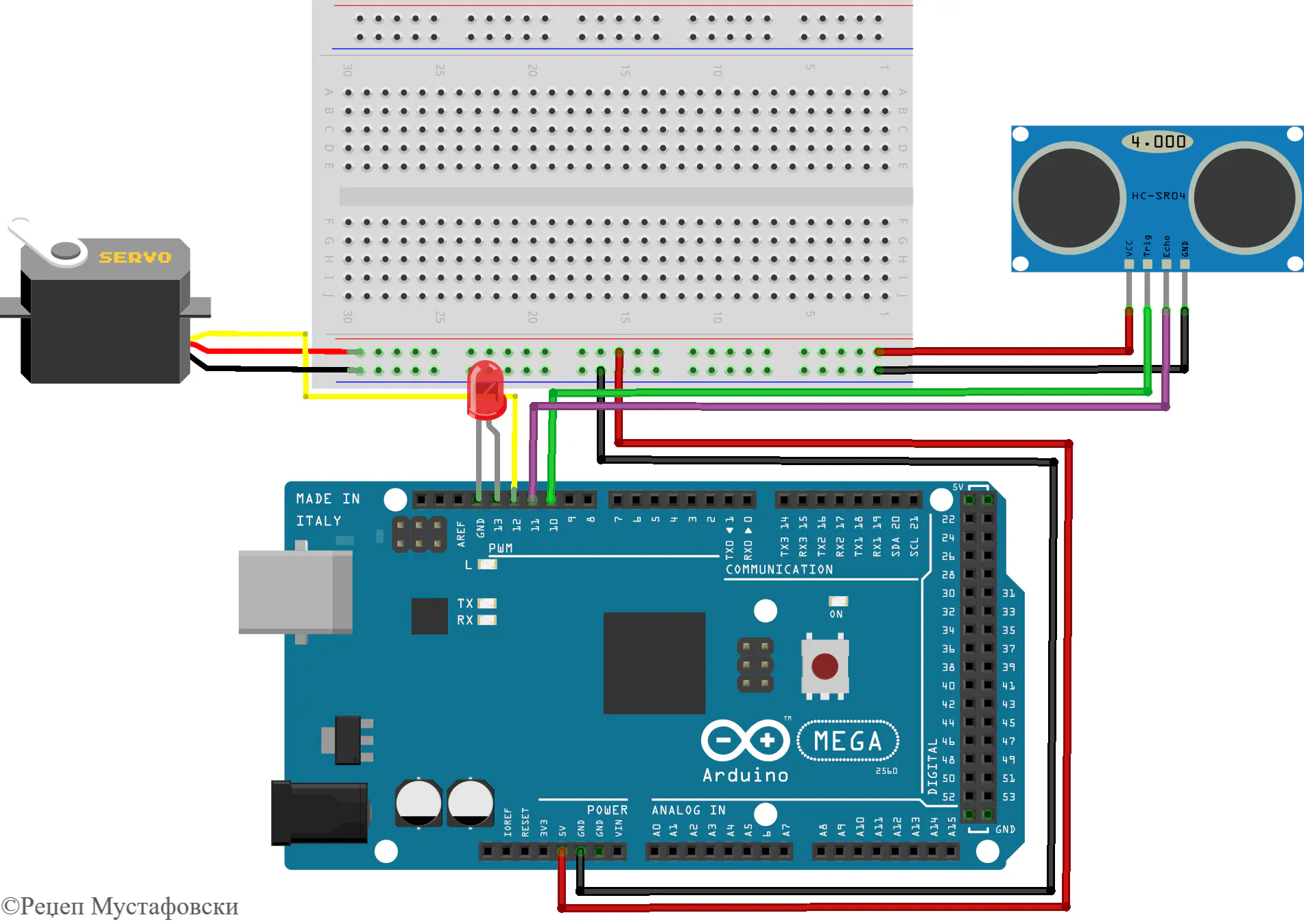### SCHEMATICS

Arduino Mega 2560 Sketch of connecting the components

## Code

### Arduino IDE code

C/C++
Copy and paste the code in Arduino IDE.
```// Includes the Servo library
#include <Servo.h>.

// Defines Tirg and Echo pins of the Ultrasonic Sensor and pin of LED
const int trigPin = 10;
const int echoPin = 11;
const int LED = 13;
// Variables for the duration and the distance
long duration;
int distance;

Servo myServo; // Creates a servo object for controlling the servo motor

void setup() {
pinMode(trigPin, OUTPUT); // Sets the trigPin as an Output
pinMode(echoPin, INPUT); // Sets the echoPin as an Input
pinMode(13, OUTPUT); // Sets the LED as an Input
Serial.begin(115200);
myServo.attach(12); // Defines on which pin is the servo motor attached
}
void loop() {
// rotates the servo motor from 15 to 165 degrees
for(int i=15;i<=165;i++){
myServo.write(i);
delay(30);
distance = calculateDistance();// Calls a function for calculating the distance measured by the Ultrasonic sensor for each degree

Serial.print(i); // Sends the current degree into the Serial Port
Serial.print(","); // Sends addition character right next to the previous value needed later in the Processing IDE for indexing
Serial.print(distance); // Sends the distance value into the Serial Port
Serial.print("."); // Sends addition character right next to the previous value needed later in the Processing IDE for indexing
}
// Repeats the previous lines from 165 to 15 degrees
for(int i=165;i>15;i--){
myServo.write(i);
delay(30);
distance = calculateDistance();
Serial.print(i);
Serial.print(",");
Serial.print(distance);
Serial.print(".");
}
}
// Function for calculating the distance measured by the Ultrasonic sensor
int calculateDistance(){

digitalWrite(trigPin, LOW);
delayMicroseconds(2);
// Sets the trigPin on HIGH state for 10 micro seconds
digitalWrite(trigPin, HIGH);
delayMicroseconds(10);
digitalWrite(trigPin, LOW);
duration = pulseIn(echoPin, HIGH); // Reads the echoPin, returns the sound wave travel time in microseconds
distance= duration*0.034/2;
if (distance < 40)
{
digitalWrite(13, HIGH);
delay(10);
}
else
{
digitalWrite(13, LOW);
delay(10);
}
return distance;
}
```

### Processing PDE code

C/C++
Copy and paste the code in Processing PDE.
```/*   Arduino Radar Project
*
*   Updated version. Fits any screen resolution!
*   Just change the values in the size() function,
*
*  by Dejan Nedelkovski, updated by Rexhep Mustafovski
*
*/
import processing.serial.*; // imports library for serial communication
import java.awt.event.KeyEvent; // imports library for reading the data from the serial port
import java.io.IOException;
Serial myPort; // defines Object Serial
// defubes variables
String angle="";
String distance="";
String data="";
String noObject;
float pixsDistance;
int iAngle, iDistance;
int index1=0;
int index2=0;
int pravec=1;
PFont orcFont;
void setup() {

//size (1200, 950); // ***CHANGE THIS TO YOUR SCREEN RESOLUTION***

fullScreen();   //   *********************  Size e zameneto s fullScreen    taka da avtomatski ke se zadadat height i with

Razmer = (height-height*0.21)*0.025; // cm pretvarame vo pikseli so formulata:  x*Razmer = x * ((height-height*0.25)*0.025);       Dolzina[vo cm] * (RabotnaVisinaEkranVoPixeli * 1/40)   max=40cm

smooth();
myPort = new Serial(this,"COM3", 115200); // starts the serial communication
myPort.bufferUntil('.'); // reads the data from the serial port up to the character '.'. So actually it reads this: angle,distance.

//************************************************************ dopolna
//iAngle = 0;
//iDistance = 25;
//frameRate(100);

//************************************************************

}
void draw() {

fill(98,245,31);
textFont(orcFont);
// simulating motion blur and slow fade of the moving line
noStroke();
fill(0,5);
rect(0, 0, width, height-height*0.065);

fill(98,245,31); // green color
// calls the functions for drawing the radar
drawLine();
drawObject();
drawText();

//************************************************************ dopolna za Avtomatski test na iscrtuvanjeto

//iAngle = iAngle+pravec;
// if (iAngle==0) {
//  pravec=pravec*(-1);
//   }
//  else if (iAngle==180)
//  {
//  pravec=pravec*(-1);
//  }

//************************************************************
}
void serialEvent (Serial myPort) { // starts reading data from the Serial Port
// reads the data from the Serial Port up to the character '.' and puts it into the String variable "data".
data = data.substring(0,data.length()-1);

index1 = data.indexOf(","); // find the character ',' and puts it into the variable "index1"

// angle= (data.substring(0, index1)); // read the data from position "0" to position of the variable index1 or thats the value of the angle the Arduino Board sent into the Serial Port
//distance= data.substring(index1+1, data.length()); // read the data from position "index1" to the end of the data pr thats the value of the distance

angle= trim(data.substring(0, index1));                     // dodadena funkcija trim za da gi otvrli praznite mesta
distance= trim(data.substring(index1+1, data.length()));    // dodadena funkcija trim za da gi otvrli praznite mesta

// converts the String variables into Integer
iAngle = int(angle);
iDistance = int(distance);
}
pushMatrix();
translate(width/2,height-height*0.074); // moves the starting coordinats to new location
noFill();
strokeWeight(2);
stroke(98,245,31);
// draws the arc lines

arc(0,0,2*40*Razmer,2*40*Razmer,PI,TWO_PI);     //      Moi novi formuli  so krugovi na 10, 20, 30 i 40 cm
arc(0,0,2*30*Razmer,2*30*Razmer,PI,TWO_PI);
arc(0,0,2*20*Razmer,2*20*Razmer,PI,TWO_PI);
arc(0,0,2*10*Razmer,2*10*Razmer,PI,TWO_PI);

//arc(0,0,(width-width*0.0625),(width-width*0.0625),PI,TWO_PI);
//arc(0,0,(width-width*0.27),(width-width*0.27),PI,TWO_PI);            //  Originalni formuli
//arc(0,0,(width-width*0.479),(width-width*0.479),PI,TWO_PI);
//arc(0,0,(width-width*0.687),(width-width*0.687),PI,TWO_PI);

// draws the angle lines
line(-width/2,0,width/2,0);
popMatrix();
}
void drawObject() {
pushMatrix();
translate(width/2,height-height*0.074); // moves the starting coordinats to new location
strokeWeight(9);
stroke(255,10,10); // red color

pixsDistance = iDistance*Razmer; // converts the distance from the sensor from cm to pixels
// limiting the range to 40 cms
if(iDistance<40){
// draws the object according to the angle and the distance

}
popMatrix();
}
void drawLine() {
pushMatrix();
strokeWeight(9);
stroke(30,250,60);
translate(width/2,height-height*0.074); // moves the starting coordinats to new location
popMatrix();
}
void drawText() { // draws the texts on the screen

pushMatrix();
if(iDistance>40) {
noObject = "Out of Range";
}
else {
noObject = "In Range";
}
fill(0,0,0);
noStroke();
rect(0, height-height*0.0648, width, height);
fill(98,245,31);
textSize(25);

//text("10cm",width-width*0.41,height-height*0.0833);
//text("20cm",width-width*0.3,height-height*0.0833);
//text("30cm",width-width*0.18,height-height*0.0833);
//text("40cm",width-width*0.06,height-height*0.0833);

text("10cm",width/2+7*Razmer,height-height*0.0833);
text("20cm",width/2+17*Razmer,height-height*0.0833);
text("30cm",width/2+27*Razmer,height-height*0.0833);
text("40cm",width/2+37*Razmer,height-height*0.0833);

textSize(40);
text("Object: " + noObject, width-width*0.875, height-height*0.0277);
text("Angle: " + iAngle +" ", width-width*0.48, height-height*0.0277);
text("Distance: ", width-width*0.26, height-height*0.0277);
if(iDistance<40) {
text("        " + iDistance +" cm", width-width*0.225, height-height*0.0277);
}
textSize(25);
fill(98,245,60);
text("30",0,0);
resetMatrix();
text("60",0,0);
resetMatrix();
text("90",0,0);
resetMatrix();
text("120",0,0);
resetMatrix();